ROC and AUC

Example Codes:

Contingency Table

A contingency table (also known as a cross tabulation or crosstab) is a type of table in a matrix format that displays the (multivariate) frequency distribution of the variables. They provide a basic picture of the interrelation between two variables and can help find interactions between them.

In reality, we frequently use it to analyze the relationship between treatment and event.

 Event No Event Treatment a b Control c d

Terms Used

Odds Ratio (OR): The odds ratio is the ratio of the odds of an event in the Treatment group to the odds of an event in the control group.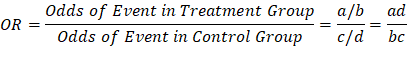Relative Risk (RR): The relative risk (RR) of an event is the likelihood of its occurrence after exposure to a risk variable as compared with the likelihood of its occurrence in a control or reference group.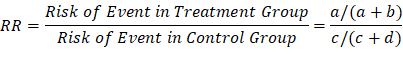Confusion Matrix

A confusion matrix, also known as an error matrix, is a specific table layout that allows visualization of the performance of an algorithm. It is a special kind of contingency table, with two dimensions ("actual" and "predicted"), and identical sets of "classes" in both dimensions (each combination of dimension and class is a variable in the contingency table).

The Layout of the confusion matrix is as follow:

 Predicted Condition Total population = P + N Positive (PP) Negative (PN) Actual condition Positive (P) True Positive (TP) False Negative (FN) Negative (N) False Positive (FP) True Negative (TN)

1.     True Positive (TP): A test result that correctly indicates the presence of a condition or characteristic.

2.     True Negative (TN): A test result that correctly indicates the absence of a condition or characteristic.

3.     False Positive (FP): A test result which wrongly indicates that a particular condition or attribute is present.

4.     False Negative (FN): A test result which wrongly indicates that a particular condition or attribute is absent.

Terms Used

TPR/Sensitivity: True positive rate, measures the proportion of positives that are correctly identified(correctly identify those with a disease).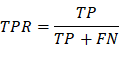TNR/Specificity: True negative rate, measures the proportion of negatives

that are correctly identified (correctly identify those without a disease).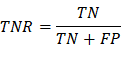FPR/Type I Error: False positive rate, measures the proportion of negatives that are wrongly categorized as positives. (The probability of rejecting the null hypothesis when it’s true)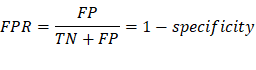FNR/Type II Error: False negative rate, measures the proportion of positives that are wrongly categorized as negatives. (The probability of accepting the null hypothesis when it’s false)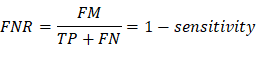PPV/Precision: Positive predictive value, the probability that following a positive test result, that individual will truly be positive.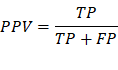NPV: Negative predictive value, the probability that following a negative test result, that individual will truly be negative.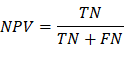R Example

#Install required packages

install.packages('caret')

install.packages('epitools')

#Import required library

library(caret)

#Creates vectors having data points

expected_value <- factor(c(1,0,1,0,1,1,1,0,0,1))

predicted_value <- factor(c(1,0,0,1,1,1,0,0,0,1))

#Creating confusion matrix

example <- confusionMatrix(data=predicted_value, reference = expected_value)

#Display results (including Sensitivity, Specificity, PPV, NPV)

example

#Simpler way - confusion matrix alone

tab = table(expected_value,predicted_value)

tab

# OR and RR

epitools::oddsratio(tab, method = 'wald')

epitools::riskratio(tab, method = 'wald')

SAS Example

data predicts;

input expect predict;

datalines;

1 1

0 0

1 0

0 1

1 1

1 1

1 0

0 0

0 0

1 1

;

proc freq data=predicts;

tables expect*predict / chisq relrisk senspec;

run;

ROC and AUC

The ROC curve (relative operating characteristic curve) is a probability curve created by plotting the TPR (sensitivity) against FPR (1-specificity) at various thresholding with FPR on the x axis and TPR on the y axis. It is a performance measurement for the classification problems.

AUC (area under the ROC curve) represents the degree or measure of separability. It measures the model’s capability of distinguishing between classes. The higher the AUC, the better the model is to classify 0 classes as 0 and 1 classes as 1(separability).

Interpretation:

-        AUC close to 1: The model has a good measure of separability, indicating a perfect model to distinguish between positive(disease) and negative(no-disease) classes.

-        AUC close to 0: The model has really bad separability. Moreover, it is identifying 0 classes as 1 and 1 classes as 0.

-        AUC = 0.5: the model has no capacity to separate classes, the prediction will be close to random guess.

-        AUC = 0.8, there is a 80 percent chance for the model to correctly distinguish between classes

In general, a rule of thumb of interpreting the accuracy from AUC and ROC is that a test with an area greater than 0.9 has high accuracy, while 0.7–0.9 indicates moderate accuracy, 0.5–0.7, low accuracy and 0.5 a chance result.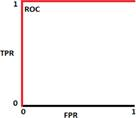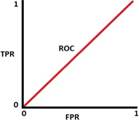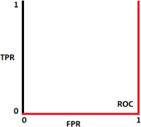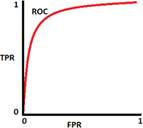AUC = 1            AUC = 0.5           AUC = 0            AUC = 0.8

R Example

library(ROCR)

library(mlbench)

# recoding the categorical variables into class 1 and class 0

data(BreastCancer)

df = data.frame(BreastCancer)

df\$type <- ifelse (df\$ Class == "benign", 1, 0)

#build training and test sets from the dataset

index = sample(1 : nrow(df), size = 0.6 * nrow(df))

train = df[index, ]

test = df[-index,]

#fitting a logistic regression model

model = glm(type ~ Cell.size + Cell.shape + Epith.c.size, data=train,

#predicting the test data

pred = predict(model, test, type="response")

pred = prediction(pred, test\$type) #plotting ROC

roc = performance(pred, "tpr", "fpr")

plot(roc, colorize = T, lwd = 2)

abline(a = 0, b = 1) #calculating AUC

auc = performance(pred, measure = "auc")

auc@y.values

SAS Example

data roc;

input alb tp totscore popind @@;

totscore = 10 - totscore;

datalines;

3.0 5.8 10 0   3.2 6.3  5 1   3.9 6.8  3 1   2.8 4.8  6 0

3.2 5.8  3 1   0.9 4.0  5 0   2.5 5.7  8 0   1.6 5.6  5 1

3.8 5.7  5 1   3.7 6.7  6 1   3.2 5.4  4 1   3.8 6.6  6 1

4.1 6.6  5 1   3.6 5.7  5 1   4.3 7.0  4 1   3.6 6.7  4 0

2.3 4.4  6 1   4.2 7.6  4 0   4.0 6.6  6 0   3.5 5.8  6 1

3.8 6.8  7 1   3.0 4.7  8 0   4.5 7.4  5 1   3.7 7.4  5 1

3.1 6.6  6 1   4.1 8.2  6 1   4.3 7.0  5 1   4.3 6.5  4 1

3.2 5.1  5 1   2.6 4.7  6 1   3.3 6.8  6 0   1.7 4.0  7 0

3.7 6.1  5 1   3.3 6.3  7 1   4.2 7.7  6 1   3.5 6.2  5 1

2.9 5.7  9 0   2.1 4.8  7 1   2.8 6.2  8 0   4.0 7.0  7 1

3.3 5.7  6 1   3.7 6.9  5 1   3.6 6.6  5 1

;

ods graphics on;

proc logistic data=roc plots(only)=roc;

LogisticModel: model popind(event='0') = alb tp totscore;

output out=LogiOut predicted=LogiPred;       /* output predicted value, to be used later */

run;

References

Karimollah H.T(2013) Receiver Operating Characteristics(ROC) Curve Analy- sis for Medical Diagnostic Test Evaluation. Caspian J Intern Med 2013 Spring;4(2) 627-635 https://cran.r-project.org/web/packages/ROCR/ROCR.pdf

Anthony K Akobeng(2007) Understanding diagnostic tests 3: receiver operating characteristic curves https://doi.org/10.1111/j.1651-2227.2006.00178.x